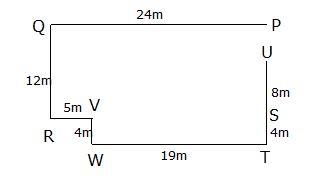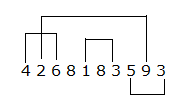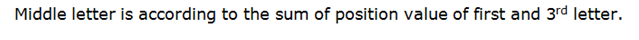# RRB Clerk Prelims Reasoning Ability Questions 2021 (Day-02)

Dear Aspirants, Our IBPS Guide team is providing new series of Reasoning Questions for RRB Clerk Prelims 2021 so the aspirants can practice it on a daily basis. These questions are framed by our skilled experts after understanding your needs thoroughly. Aspirants can practice these new series questions daily to familiarize with the exact exam pattern and make your preparation effective.

Start Quiz

Number series

Direction (1-5): Study the following information carefully and answer the below questions

943 521 476 853 625

1) If the numbers are written in ascending order within the number, which of the following number will become the second-highest number?

A.943

B.521

C.476

D.853

E.625

2) If the first digit of the lowest number is multiplied with the third digit of the second-highest number then which of the following will be the resultant?

A.48

B.24

C.18

D.12

E.None of the above

3) If the position of first and last digits is interchanged how many numbers will be divisible by three?

A.1

B.2

C.3

D.4

E.None

4) In each three digit number, the first digit is added to the last digit, then the results should be subtracted from the second digit then which of the following yields the highest number?

A.943

B.521

C.476

D.853

E.625

5) Find the odd one out

A.BDG

B.FHK

C.LNQ

D.RTW

E.UWY

Direction sense

Direction (6-8): Study the following information carefully and answer the below questions.

Point P is 24m east of point Q. Point R is 12m south of point Q. Point W is 19m west of point T which is 4m south of point S. Point V is 5m east of point R. Point U is 8m north of point S. Point V is 4m north of point W.

6) In which direction is point U with respect to point R?

A. North

B. South

C. North-east

D. South-west

E. None of these

7) What is the shortest distance between point V and point Q?

A.18m

B.16m

C.14m

D.13m

E.None of these

8) How far and in which direction is point T with respect to point P?

A.16m, north

B.12m, south

C.16m, south

D.12m, north

E.None of these

Miscellaneous

9) How many pairs of digits are there in the number “4268183593” as in between digits in the numerical series in both forward and backward directions?

A.1

B.2

C.3

D.4

E.None

10) Find the odd one out from the following term.

A.HXP

B.FRL

C.BFD

D.DMH

E.EOJ

943 521 476 853 625

349 125 467 358 256

943 521 476 853 625

3*4=12

943 521 476 853 625

349 125 674 358 526

943 521 476 853 625

8 4 3 6 9

One alphabet is between first and second letter and two alphabets are between the second and third letter according to the English alphabetical series.

Directions (6-8) :